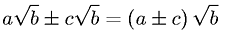Equations > Algebra > Surds > SURD Addition and SubtractionLatex Code:

MathML Code:

 $a\sqrt{b}±c\sqrt{b}=\left(a±c\right)\sqrt{b}$

MathType 5.0: